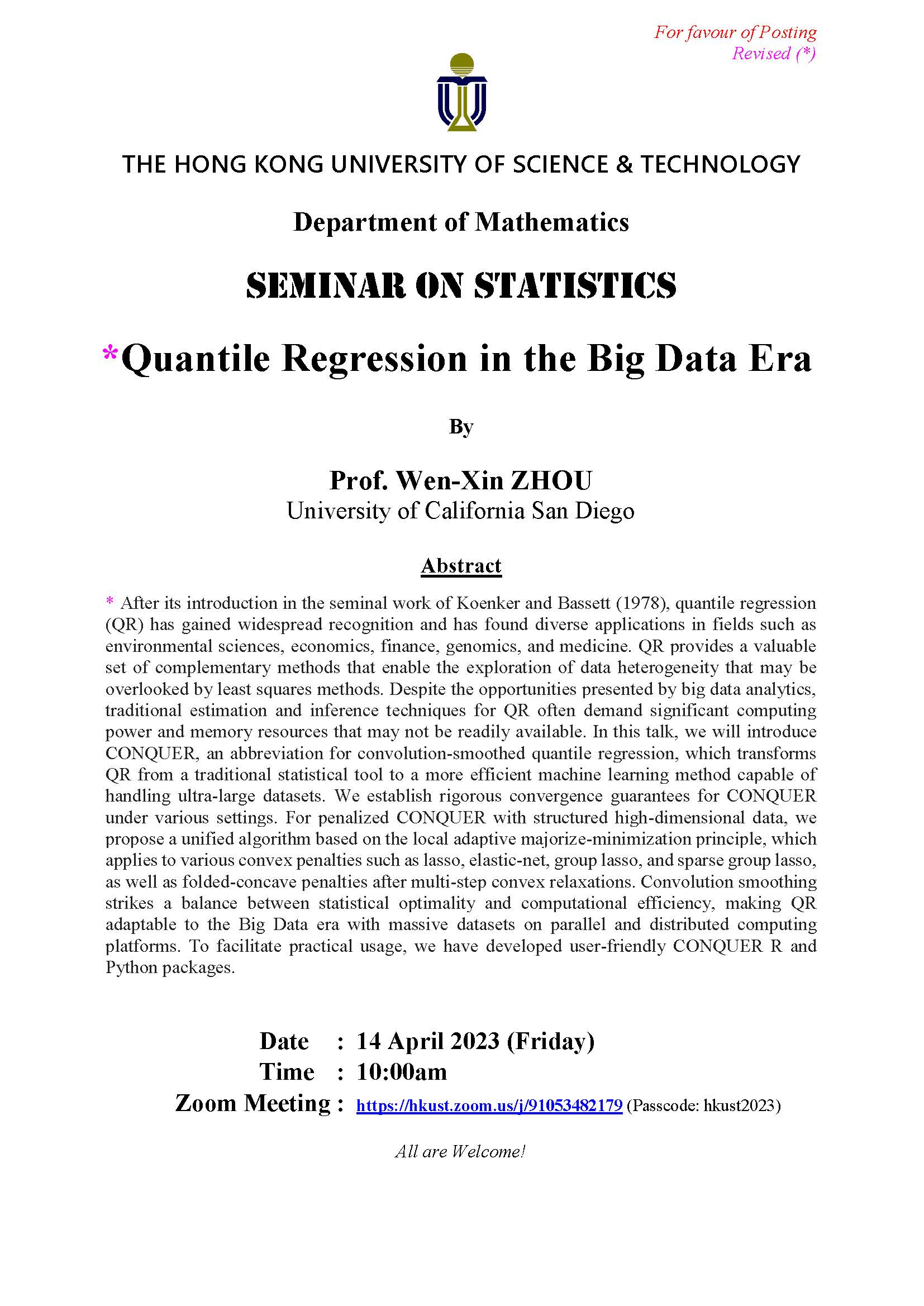Expected Shortfall (ES), also known as superquantile or Conditional Value-at-Risk, has been recognized as an important measure in risk analysis and stochastic optimization. In finance, it refers to the conditional expected return of an asset given that the return is below some quantile of its distribution. In this work, we consider a joint regression framework that simultaneously models the conditional quantile and ES of a response variable given a set of covariates, for which the state-of-the-art approach is based on minimizing a joint loss function that is non-differentiable and non-convex.

Motivated by the idea of using Neyman-orthogonal scores to reduce sensitivity with respect to nuisance parameters, we propose a statistically robust and computationally efficient two-step procedure for fitting joint quantile and ES regression models. With increasing covariate dimensions, we establish explicit non-asymptotic bounds on estimation and Gaussian approximation errors, which lay the foundation for statistical inference. Under high-dimensional sparse models, we consider a lasso-penalized two-step approach and its robust counterpart, and propose debased estimators for inference. We further discuss a more general weighted-average quantile regression framework, including ES regression as a special case. This talk is based on joint works with Xuming He, Kean Ming Tan and Shushu Zhang.

4月14日
10am - 11amhttps://hkust.zoom.us/j/91053482179 (Passcode: hkust2023)

Prof. Wen-Xin ZHOU
University of California San Diego

Department of Mathematics

Alumni, Faculty and staff, PG students, UG students6月26日

Department of Mathematics - Seminar on PDE - Some recent results on asymptotically Poincare-Einstein manifolds
Poincar-Einstein manifolds are a class of noncompact Riemannian manifolds with a well- defined boundary at infinity. They appear as the framework of AdS/CFT correspondence in string the...6月23日

Department of Mathematics - Seminar on Applied Mathematics - Deep Particle: learning invariant measure by a deep neural network minimizing Wasserstein distance on data generated from an interacting particle method
High dimensional partial differential equations (PDE) are challenging to compute by traditional mesh-based methods especially when their solutions have large gradients or concentrations...